Start typing, then use the up and down arrows to select an option from the list.# Hess's Law & Equilibrium Constant K

Jules Bruno
400views
8
Hey, guys. So here we have to isolate this equation. So what we're gonna do is we're gonna go one by one and see what we find each one of these compounds. So ch four, where do we see ch four? Here's ch four and matches perfectly with the one that we see up here. Which means we do not manipulate or touch this equation next. 02 Or do we co two? We find it in two places, which means that we should not do anything. If you find it in more than one place, skip it and move on. Co two, Where do we see co two? We see CO two right here again. They match perfectly with each other, which means we should not attempt to touch this equation either. And then finally, we have two moles of H 12 liquid. Here goes H two a liquid. But we have two issues. First, we needed to be a product like it is here and also we need two moles of it. So what to changes? Do we need to do well, we're gonna have to reverse this reaction in order to make each to a liquid a product and we're gonna have to multiply by two. So that has two moles of it. So when we do that, we get to H 20 gas gives me to H liquid. Remember, when we reverse the reaction, we get the reciprocal of K. So it's gonna become 1/6 0.12 times 10 to the negative four and I multiplied by two. When you multiply by a number, it becomes the power when you punch this into your calculator. So first do one divided by this number 6.12 times 10 to the negative four. Make sure this is in parentheses. When you do it, it should give you 1633. And then you square that answer which gives you 2.66991 times 10 to the six. So now this equation is gone because it's been manipulated to this new one same thing with cat and then we cross out our intermediates soon cancels out Well, we have two moles of H 20 gas which cancels out with these two moles of H 20 gas as products. So this here is called formaldehyde, which cancels out with this formaldehyde, which is a product, Remember, intermediates look alike, except one will be a reactive and the other will be a product. Now, those are the only intermediates. Everything else comes down. So ch four comes down and then these two old tools are on the same side. When they're on the same side, they don't cancel out. They add up this co two gas comes down and then are two moles of H 20 liquid come down. So we isolated the equation we needed so to figure out this K C four. Now all we do is we multiply K C one times k c. Two times, Casey three which we found earlier. When you multiply all three together, that will give you your K up 1.29 times 10 to the six. So this will be our answer. And this will be the missing equilibrium constant for our equation.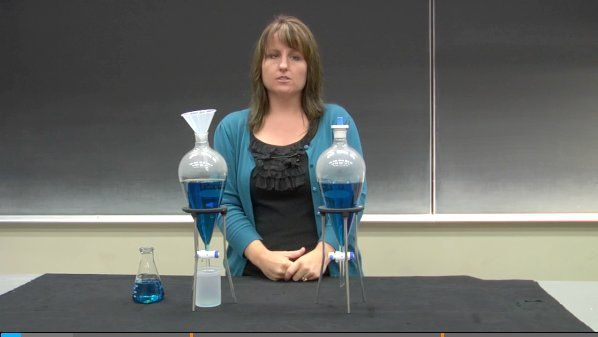3:49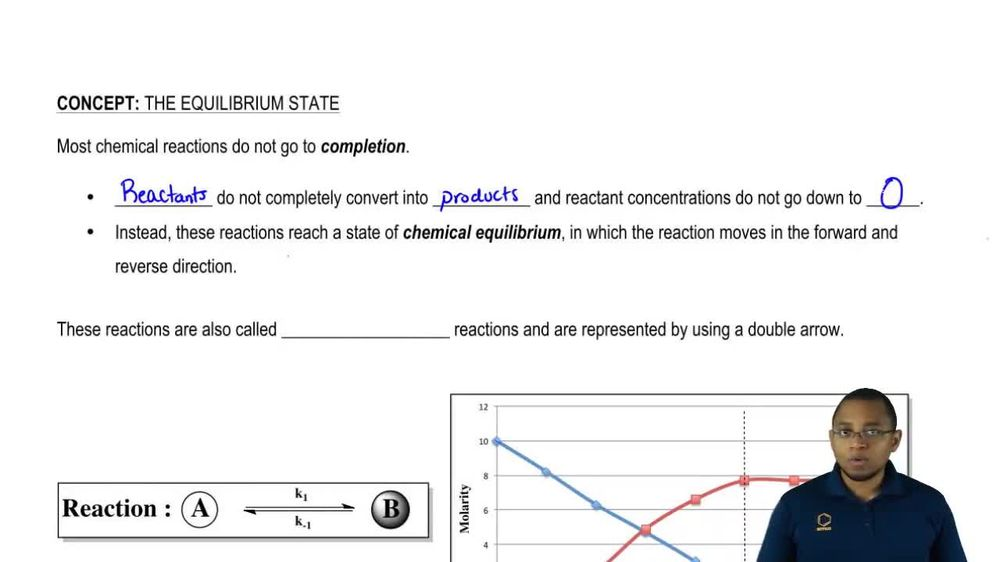03:42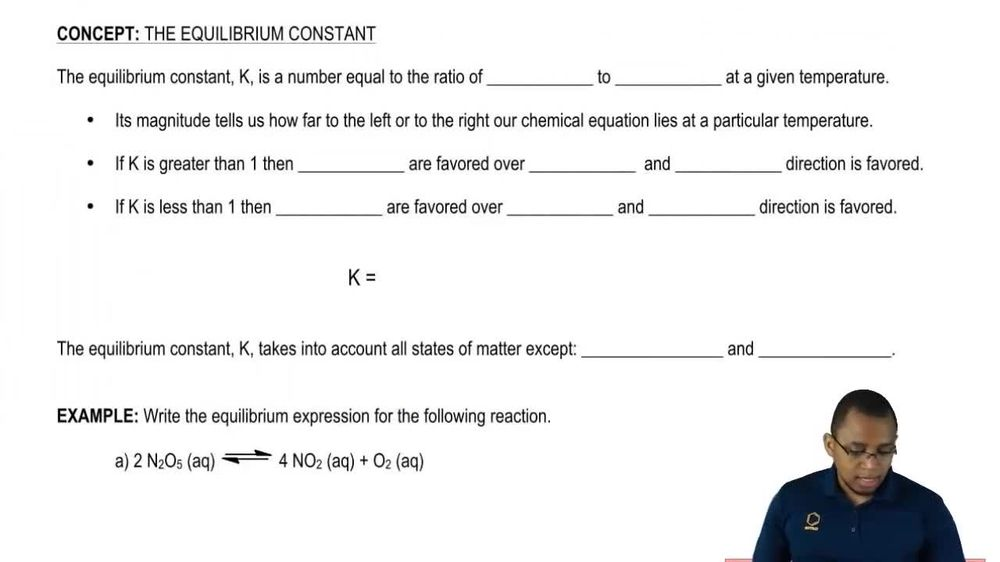02:50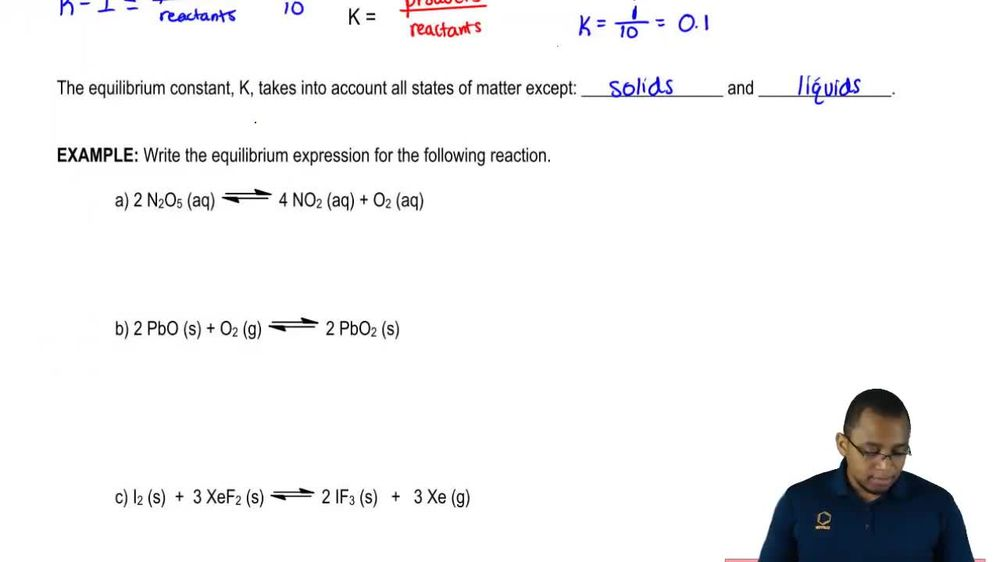03:43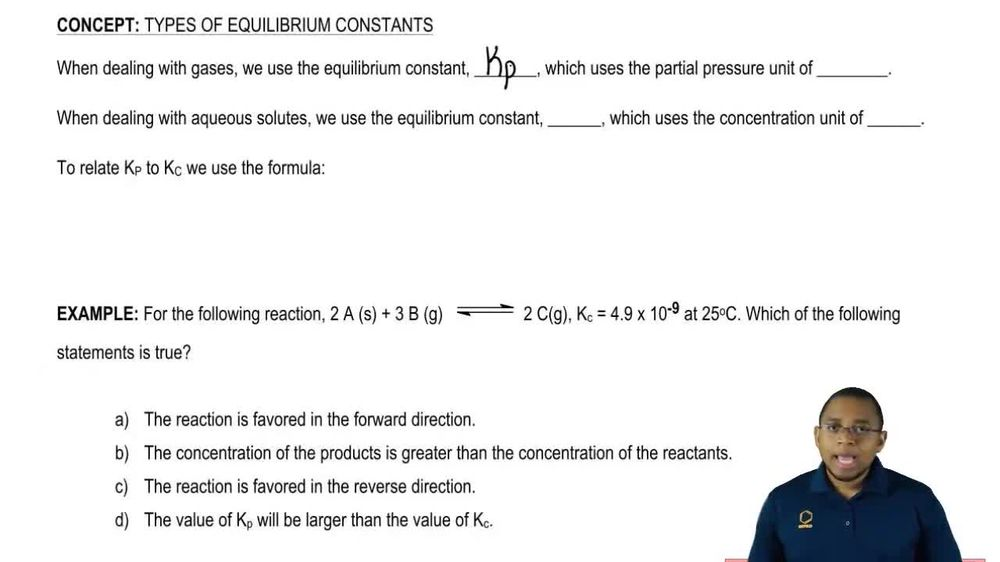03:01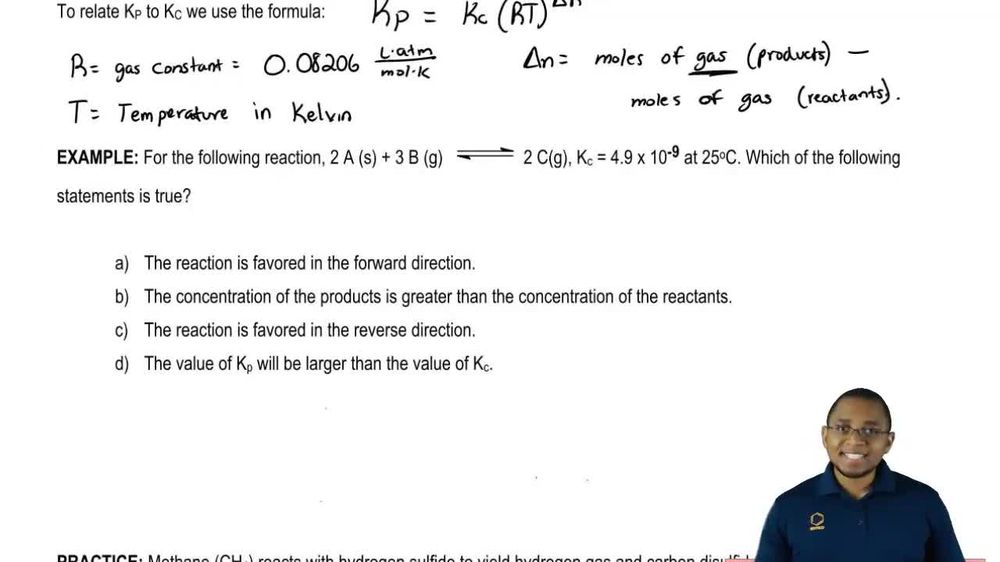03:35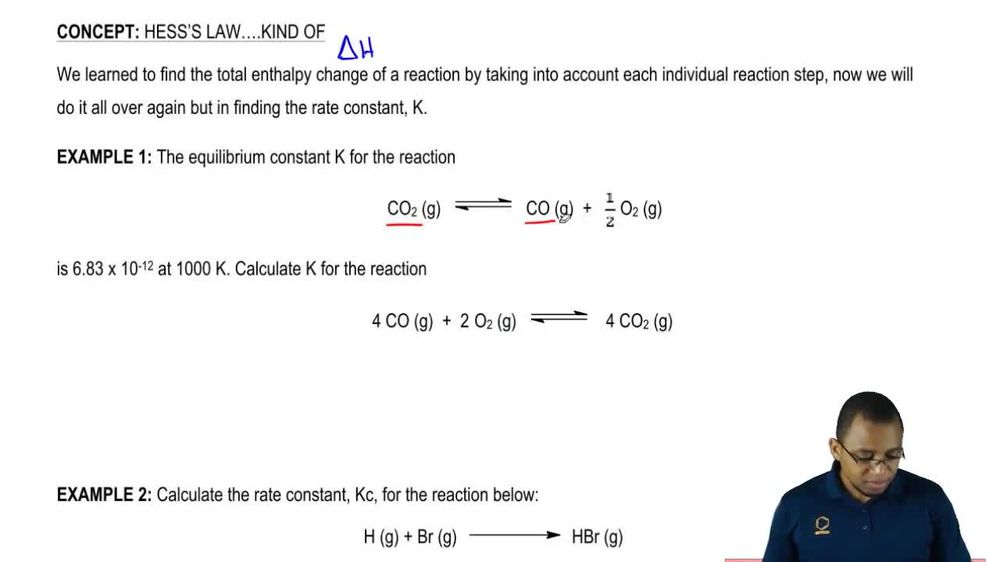03:58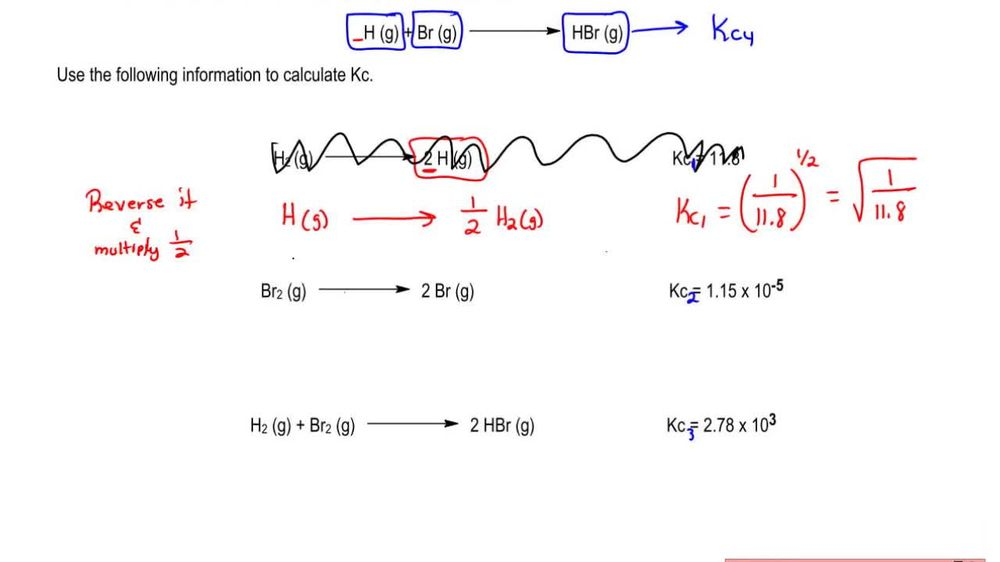06:46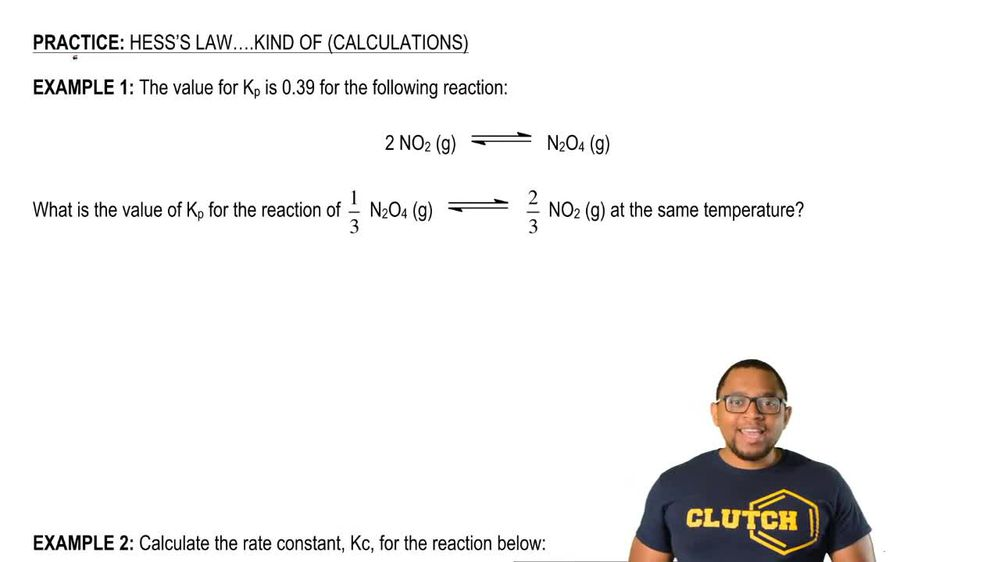03:39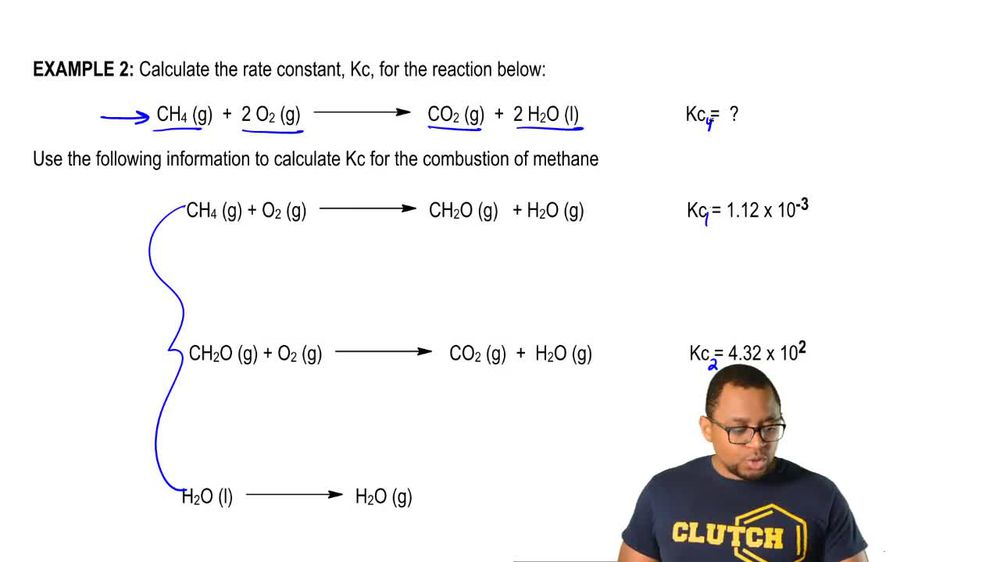03:28